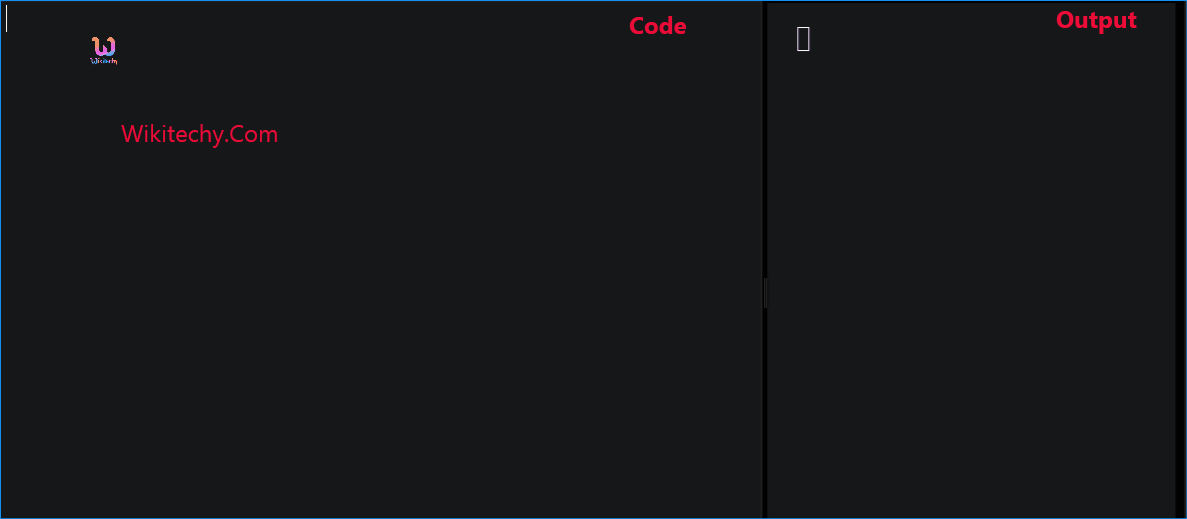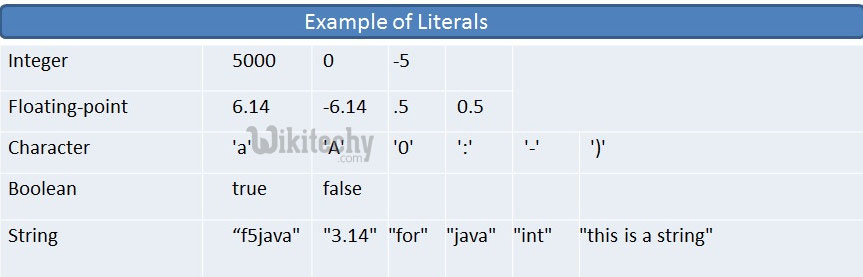# C++ Constants | Constants - Learn C++ - C++ Tutorial - C++ programmingLearn c++ - c++ tutorial - c++ constants - c++ examples - c++ programs

## What is Constant in C++?

• Constants refer to fixed values that the program may not alter and they are called literals.
• Constants can be of any of the basic data types and can be divided into Integer Numerals, Floating-Point Numerals, Characters, Strings and Boolean Values.
• Again, constants are treated just like regular variables except that their values cannot be modified after their definition.
•learn c++ tutorials - example-of-literals Example

## Integer literals

• An integer literal can be a decimal, octal, or hexadecimal constant.
• A prefix specifies the base or radix: 0x or 0X for hexadecimal, 0 for octal, and nothing for decimal.
• An integer literal can also have a suffix that is a combination of U and L, for unsigned and long, respectively.
• The suffix can be uppercase or lowercase and can be in any order.
•learn c++ tutorials - literals Example

• Here are some examples of integer literals:
• Following are other examples of various types of Integer literals:

## Floating-point literals

• A floating-point literal has an integer part, a decimal point, a fractional part, and an exponent part.
• You can represent floating point literals either in decimal form or exponential form.
• While representing using decimal form, you must include the decimal point, the exponent, or both and
• while representing using exponential form, you must include the integer part, the fractional part, or both.
• The signed exponent is introduced by e or E.
• Here are some examples of floating-point literals:

## Boolean literals

• There are two Boolean literals and they are part of standard C++ keywords:
• A value of true representing true.
• A value of false representing false.
• You should not consider the value of true equal to 1 and value of false equal to 0.

## Character literals

• Character literals are enclosed in single quotes. If the literal begins with L (uppercase only), it is a wide character literal (e.g., L'x') and should be stored in wchar_t type of variable . Otherwise, it is a narrow character literal (e.g., 'x') and can be stored in a simple variable of char type.
• A character literal can be a plain character (e.g., 'x'), an escape sequence (e.g., '\t'), or a universal character (e.g., '\u02C0').
• There are certain characters in C++ when they are preceded by a backslash they will have special meaning and they are used to represent like newline (\n) or tab (\t).
• Here, you have a list of some of such escape sequence codes:
Escape sequence Meaning
\\ \ character
\' ' character
\" " character
\? ? character
\b Backspace
\f Form feed
\n Newline
\r Carriage return
\t Horizontal tab
\v Vertical tab
\ooo Octal number of one to three digits
\xhh . . . Hexadecimal number of one or more digits
• Following is the example to show few escape sequence characters:
• When the above code is compiled and executed, it produces the following result:

## String literals

• String literals are enclosed in double quotes. A string contains characters that are similar to character literals:
• plain characters
• escape sequences, and
• universal characters
• You can break a long line into multiple lines using string literals and separate them using whitespaces.
• Here are some examples of string literals. All the three forms are identical strings.

## Defining Constants

• There are two simple ways in C++ to define constants:
• Using #define preprocessor.
• Using const keyword.

## The #define Preprocessor

• Following is the form to use #define preprocessor to define a constant:
• Following example explains it in detail:
• When the above code is compiled and executed, it produces the following result:

## The const Keyword

• You can use const prefix to declare constants with a specific type as follows:
• When the above code is compiled and executed, it produces the following result:
• Note that it is a good programming practice to define constants in CAPITALS.